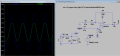Need help with Non-Inverting amplifier using single supply

AnalogKid

Joined Aug 1, 2013
9,631
A couple of things.
C3 and C1 form a 100-to-1 attenuator. Calculate the impedance of both at 1 Hz to see how this is.
C2 also is too small for a 1 Hz signal. Its impedance at 1 Hz should be less than 10% of R1, preferably less than 1%.
The connection from the 4.5V divider to the opamp + input pin should be a resistor. Start with 100K. This prevents an AC interaction between the input and the Vcc/2 decoupling capacitor C1.

ak

Bordodynov

Joined May 20, 2015
2,950
My electronic circuits are equivalent to the use of virtual land. It is simply masked. Voltage divider with two identical resistors R1 = R2 = R (e.g. 200 kohm) is an equivalent circuit consisting of a voltage source Vcc/2 and the series resistor Re = R1 || R2 = R/2. The positive pole of the equivalent source and acts as a virtual ground.
You can explain the operation of my circuits that produced by the resistors by the amount of shift voltage Vcc/2.

Yuvraj Abhimanyu

Joined Jun 10, 2015
15
The connection from the 4.5V divider to the opamp + input pin should be a resistor. Start with 100K. This prevents an AC interaction between the input and the Vcc/2 decoupling capacitor C1.
ak
I Tried putting a 100K resistor between vcc/2 and gnd, Just the thing i neededyipeeee...!!but what do u mean by "AC interaction"?

A couple of things.
C3 and C1 form a 100-to-1 attenuator. Calculate the impedance of both at 1 Hz to see how this is.
Firstly why 1Hz?
Impedence of c1 for 1Hz is 15.9K =z1(say)
Impedence of c3 for 1Hz is 1.59Meg = z2(say)
so Vo=(Vi*z2)/(z1+z2) will nearly give me 100, which means that Vo is 100 times Vi, how is this attenuating?

.
C2 also is too small for a 1 Hz signal. Its impedance at 1 Hz should be less than 10% of R1, preferably less than 1%.
why should the impedence be less than 10%? any specific reason or rule of thumb?

Yuvraj Abhimanyu

Joined Jun 10, 2015
15
I do not understand why use capacitors and limit the range of frequencies.

View attachment 87903
Thank you Bordodynov, but for my application its good to limit frequenciesAnalogKid

Joined Aug 1, 2013
9,631
you: but what do u mean by "AC interaction"?

me: Your 4.5 V source has a 10 uF filter cap; that's good. But the idea is to create a virtual ground, which means anything connected to it is "shorted" to ground, within the limits of the capacitor's frequency response. As seen below, this is a 100:1 attenuator when connected directly to the 0.1uF input cap. The 100K resistor establishes a DC value for the + input and isolates the input by 100 K ohms from "ground".

you: Firstly why 1Hz?

you: Impedence of c1 for 1Hz is 15.9K =z1(say)
Impedence of c3 for 1Hz is 1.59Meg = z2(say)
so Vo=(Vi*z2)/(z1+z2) will nearly give me 100, which means that Vo is 100 times Vi, how is this attenuating?

me: Nope. It's Vi x z1 over z1+z2 because the network's output voltage is developed across C1. Think about it - a two component passive network can *never* have gain.

you: why should the impedance be less than 10%? any specific reason or rule of thumb?

me: Yes, the 10% rule. When guesstimating component values, the first-order approximation is that something that is 10% or less than something else is basically either not there or completely there. Simple resistor circuits: 1K in series with 10K is so much closer to the 10K value than the 1K value that it's like the 1K isn't there. 1K in parallel with 10K is so much closer to the 1K value than the 10K value that the 10K is almost shorted ("swamped") by the 1K. Remember, these are approximations and are inaccurate by 10%, just like the name of the rule.

ak

Last edited:
•Yuvraj Abhimanyu

Veracohr

Joined Jan 3, 2011
763
I dont understand why!
Step back to the basic model of an opamp: Vout = A*(V+ - V-). If V+ is centered at 0V and V- is centered at 4.5V, Vout is trying to be centered at A*(-4.5V), which can't happen without a negative power supply.